# Hyperbolic Functions

Hyperbolic Functions

If you have a long rope, and you hold it from the two ends.

Do you know what is the function that gives this curve?

Hyperbolic function defines this curve and the equation of the curve is: $$\text{f(x) = a cosh}\left(\dfrac{x}{a} \right)$$In this lesson, you will learn about the hyperbolic functions. We will be covering their formulas, graphs, domain and range, and understand step by step the ways to solve problems on related topics.

You can check out the interactive examples to know more about the lesson and try your hand at solving a few interesting practice questions at the end of the page.

## Lesson Plan

 1 What Is Meant by a Hyperbolic Function? 2 Important Notes on Hyperbolic Functions 3 Challenging Questions on Hyperbolic Functions 4 Solved Examples on Hyperbolic Function 5 Interactive Questions on Hyperbolic Function

## What Is Meant by a Hyperbolic Function?

As an ordinary trigonometric function is defined for or on a circle, similarly hyperbolic function is defined for hyperbola.

In ordinary trigonometry, we were using sine, cosine, and other functions. Similarly, in hyperbola, we use sinh, cosh, and other hyperbolic functions.

As in ordinary trigonometric function, (cos θ, sin θ) forms a circle of unit radius, similarly in hyperbolic function, (cosh θ, sinh θ) forms right half of equilateral hyperbola.

They also occur in the solutions of many linear differential equations, cubic equations, and Laplace's equation(Laplace's equation is very important in the field of physics, maths, and engineering).

### The basic hyperbolic functions are:

1. Hyperbolic sine or sinh
2. Hyperbolic cosine or cosh

Apart from these two basic functions, the other 4 functions which can be derived from these basic functions are:

• hyperbolic tangent or tanh
• hyperbolic cosecant  or cosech
• hyperbolic secant or sech
• hyperbolic cotangent or coth

## What Are the Formulas of Hyperbolic Function?

The hyperbolic functions are defined through the algebraic expressions that include the exponential function (ex) and its inverse exponential functions (e-x), where e is the Euler’s constant.

Let us see all the hyperbolic functions one by one:

1. Hyperbolic sine function or Sinh $$x$$:

This is the odd part of the exponential functions.

Algebriac expression for hyperbolic sine function is:

$\text {sinh x } = \dfrac{ {(\text e^\text x - \text e^\text {-x})}}2$

2. Hyperbolic cosine function or Cosh $$x$$:

This is the even part of the exponential function.

Algebriac expression for hyperbolic cosine function is:

$\text {cosh x } = \dfrac{ {(\text e^\text x + \text e^\text {-x})}}2$

3. Hyperbolic tangent function or Tanh $$x$$:

$\text {tanh x } = \dfrac {\text { sinh x }}{ \text {cosh x}} = \dfrac{ {(\text e^\text x - \text e^\text {-x})}} {(\text e^\text x + \text e^\text {-x})}$

4. Hyperbolic cotangent $$x$$ or Cot $$x$$:

$\text {coth x } = \dfrac { \text {cosh x}} {\text { sinh x }}= \dfrac{(\text e^\text x + \text e^\text {-x})}{ {(\text e^\text x - \text e^\text {-x})}}$

5. Hyperbolic secant $$x$$ or Sec $$x$$:

$\text {sech x } = \dfrac 2 { {(\text e^\text x + \text e^\text {-x})}}$

6. Hyperbolic cosecant $$x$$ or Cosec $$x$$:
$\text {cosech x } = \dfrac 2{ {(\text e^\text x - \text e^\text {-x})}}$

## Graphical Representation of Hyperbolic Function

Graph of the hyperbolic functions are given below:

1. Hyperbolic sine function or sinh $$x$$:

Domain: R and Range: R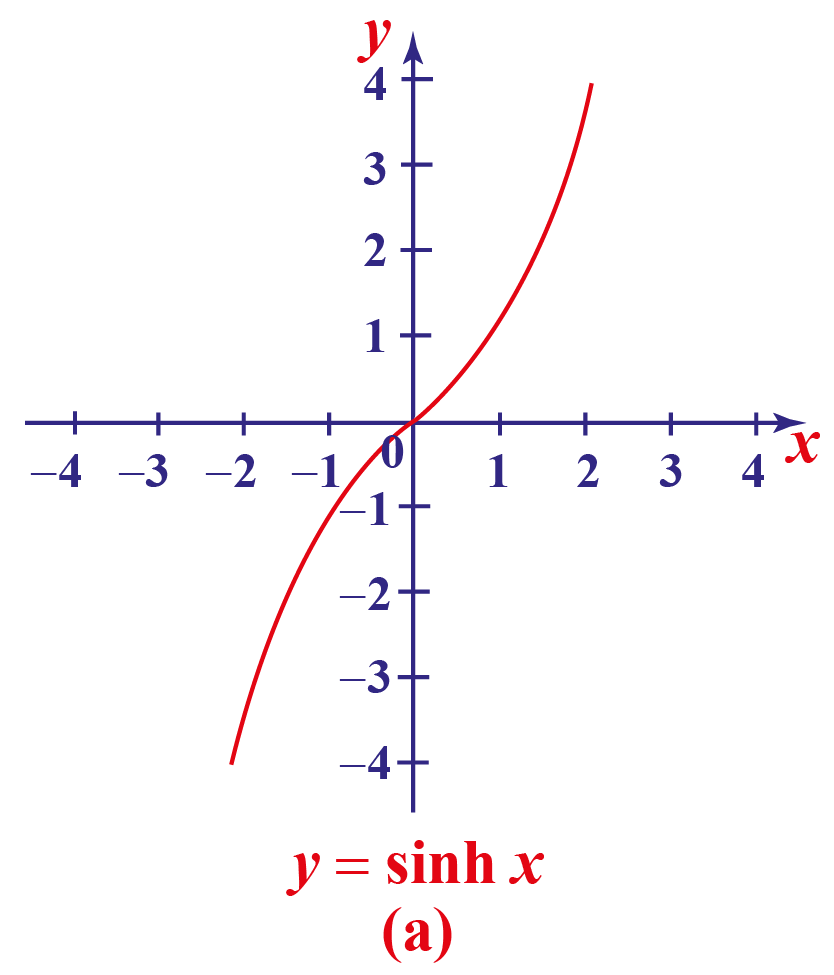2. Hyperbolic cosine function or cosh $$x$$:

Domain: R and Range: $$[1 , +\infty)$$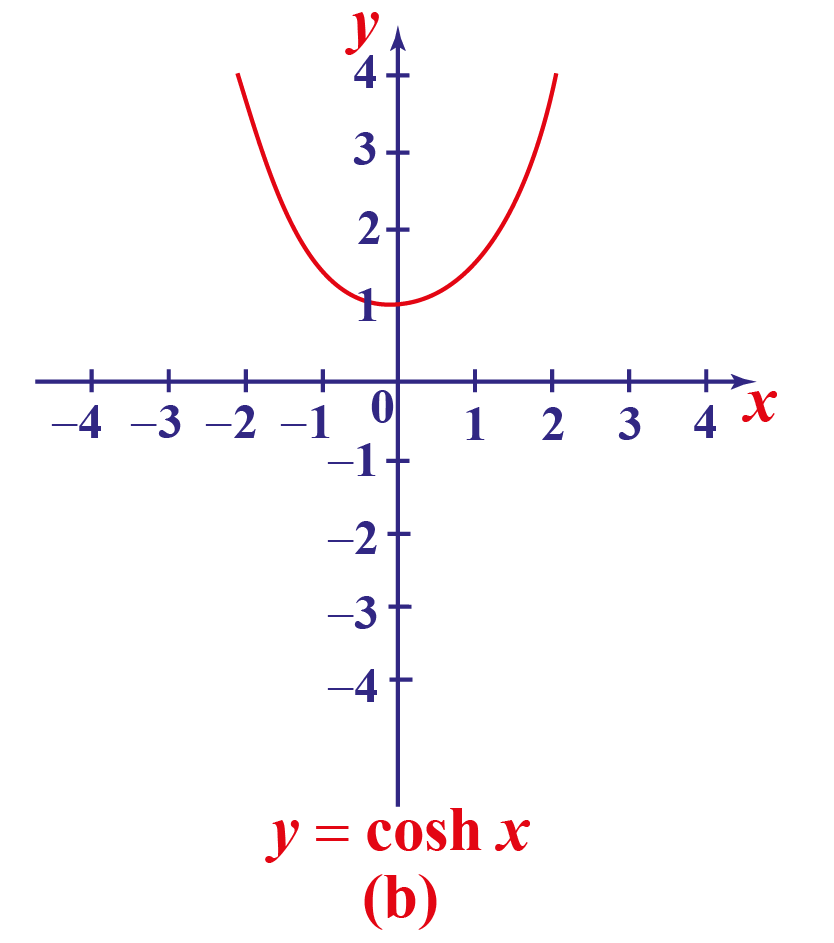3. Hyperbolic tangent function or tanh $$x$$:

Domain: R and Range: $$(-1 , + 1)$$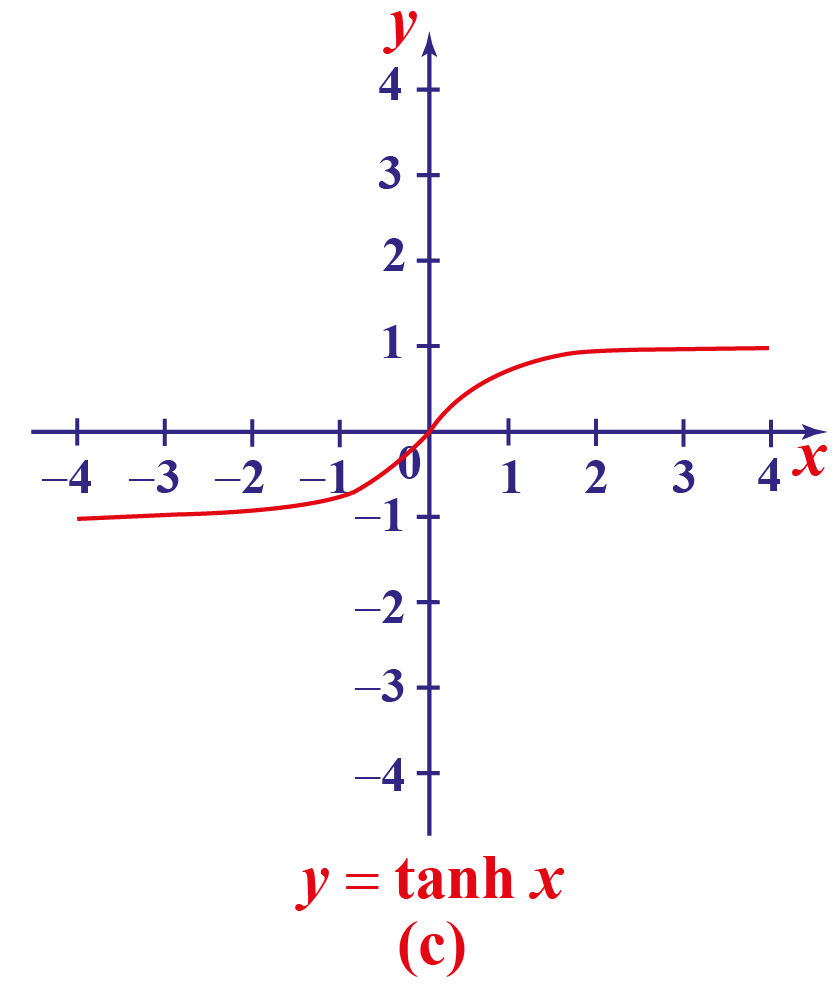4. Hyperbolic cotangent $$x$$ or coth $$x$$:

Domain: $$(-\infty , 0)$$ U $$(0 , +\infty)$$ and Range: $$(-\infty , -1)$$ U $$(1 , +\infty)$$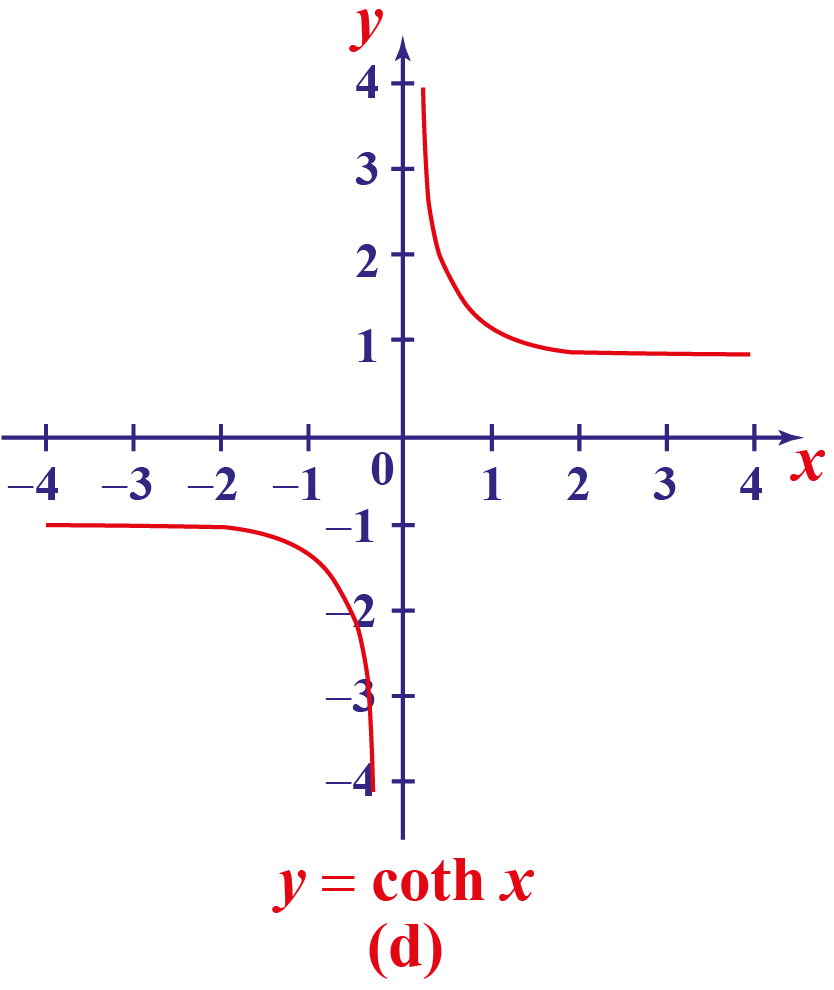5. Hyperbolic secant $$x$$ or sech $$x$$:

Domain: R and Range: (0 , 1]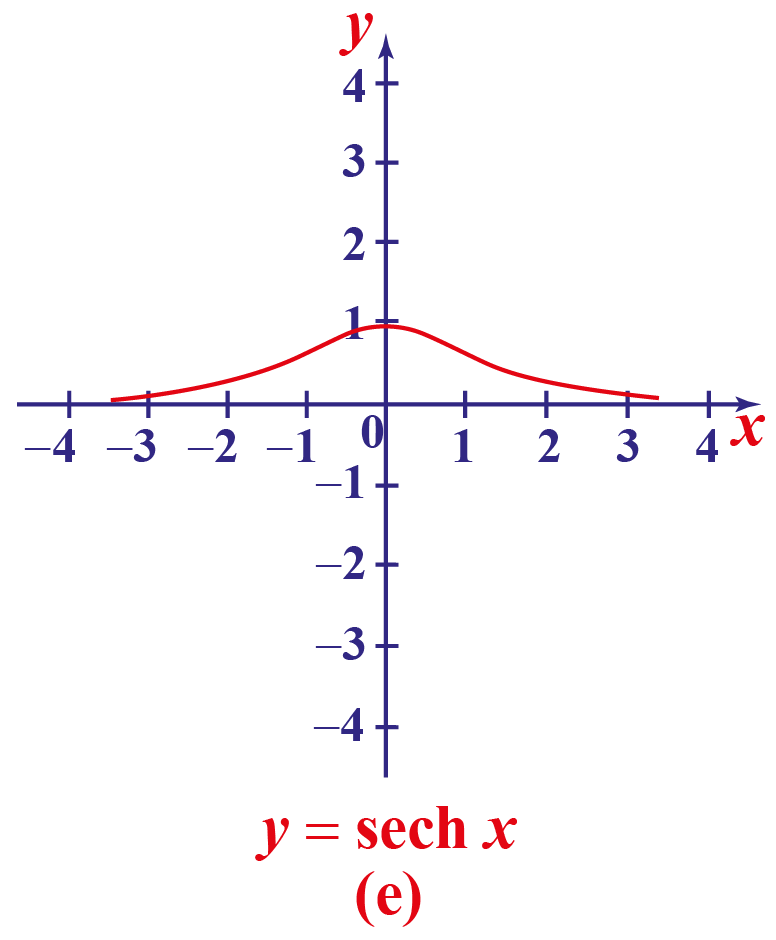6. Hyperbolic cosecant $$x$$ or cosech $$x$$:

Domain: $$(-\infty , 0)$$ U $$(0 , +\infty)$$ and Range: $$(-\infty , 0)$$ U $$(0 , +\infty)$$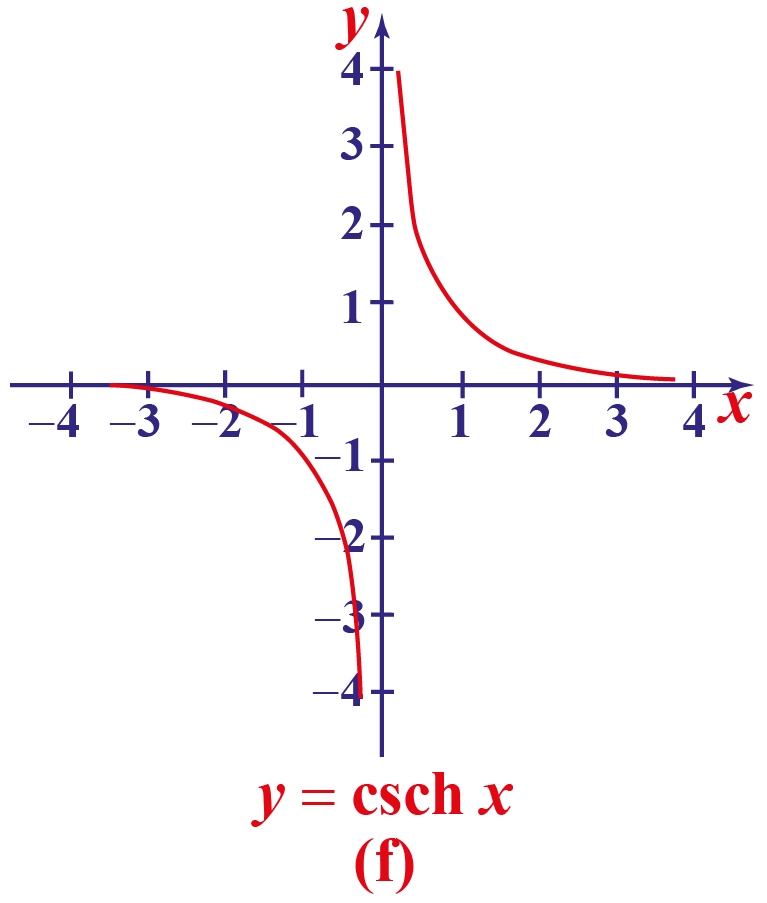## What Are the Properties of the Hyperbolic Function?

The properties of hyperbolic functions are analogous to the properties of trigonometric functions.

Examples

• $$\text {sinh (-x) = – sinh(x)}$$
• $$\text {cosh (-x) = cosh (x)}$$
• $$\text {cosh 2x = 1 + 2 sinh}^2 (\text x)$$
• $$\text {cosh 2x = cosh 2x + sinh 2x}$$
• $$\dfrac d{d \ \text x} \text{ sinh x = cosh x}$$
• $$\dfrac {\text d}{\text d \ \text x} \text{ cosh x = sinh x}$$

Hyperbolic functions can also be deduced from trigonometric functions with complex arguments:

• $$\text {sinh x = i sin(ix)}$$
• $$\text {cosh x = cos(ix)}$$
• $$\text {tanh x = - i tan(ix)}$$
• $$\text {coth x = i cot(ix)}$$
• $$\text {sech x = sec(ix)}$$
• $$\text {cosech x = i cosec(ix)}$$

## Are Hyperbolic Function Identities Similar to Trigonometric Identities?

The hyperbolic function identities are similar to trigonometric identities and can be understood better from below.

### Explanations

Osborn's rule states that, trigonometric identities can be converted into hyperbolic identities when expanded completely in terms of integral powers of sines and cosines, which includes changing sine to sinh, cosine to cosh. Sign of every term that contains a product of two sinh should be replaced.

$$\text {sinh x – sinh y} = 2\text {cosh}\dfrac {\text {(x+y)}}2 \text { sinh}\dfrac {\text {(x+y)}}2$$

$$\text {sinh x + sinh y} = 2\text {sinh}\dfrac {\text {(x+y)}}2 \text { cosh}\dfrac {\text {(x+y)}}2$$

$$\text {cosh x + cosh y} = 2\text {cosh}\dfrac {\text {(x+y)}}2 \text { cosh}\dfrac {\text {(x+y)}}2$$

$$\text {cosh x – cosh y} = 2\text {sinh}\dfrac {\text {(x+y)}}2 \text { sinh}\dfrac {\text {(x+y)}}2$$

$$2 \text {sinh x cosh y = sinh(x + y) + sinh(x - y)}$$

$$2 \text {cosh x sinh y = sinh(x + y) – sinh(x – y)}$$

$$2 \text {sinh x sinh y = cosh(x + y) – cosh(x – y)}$$

$$2 \text {cosh x cosh y = cosh(x + y) + cosh(x – y)}$$

$$\text {sinh(x ± y) = sinh x cosh x ± coshx sinh y }$$

$$\text {cosh(x ± y) = cosh x cosh y ± sinh x sinh y}$$

$$\text {tanh(x ± y) }= \ \dfrac {\text {(tanh x ± tanh y})} {(1± \text { tanh x tanh y} )}$$

$$\text {coth(x ± y)} = \dfrac {(\text {coth x coth y} ± 1) }{(\text {coth y ± coth x)} }$$

$$\text {cosh}^2 (\text x) – \text {sinh}^2 (\text x) = 1$$

$$\text {tanh}^2 (\text x) – \text {sech}^2 (\text x) = 1$$

$$\text {coth}^2 (\text x) – \text {cosech}^2 (\text x) = 1$$

More Important Topics
Numbers
Algebra
Geometry
Measurement
Money
Data
Trigonometry
Calculus